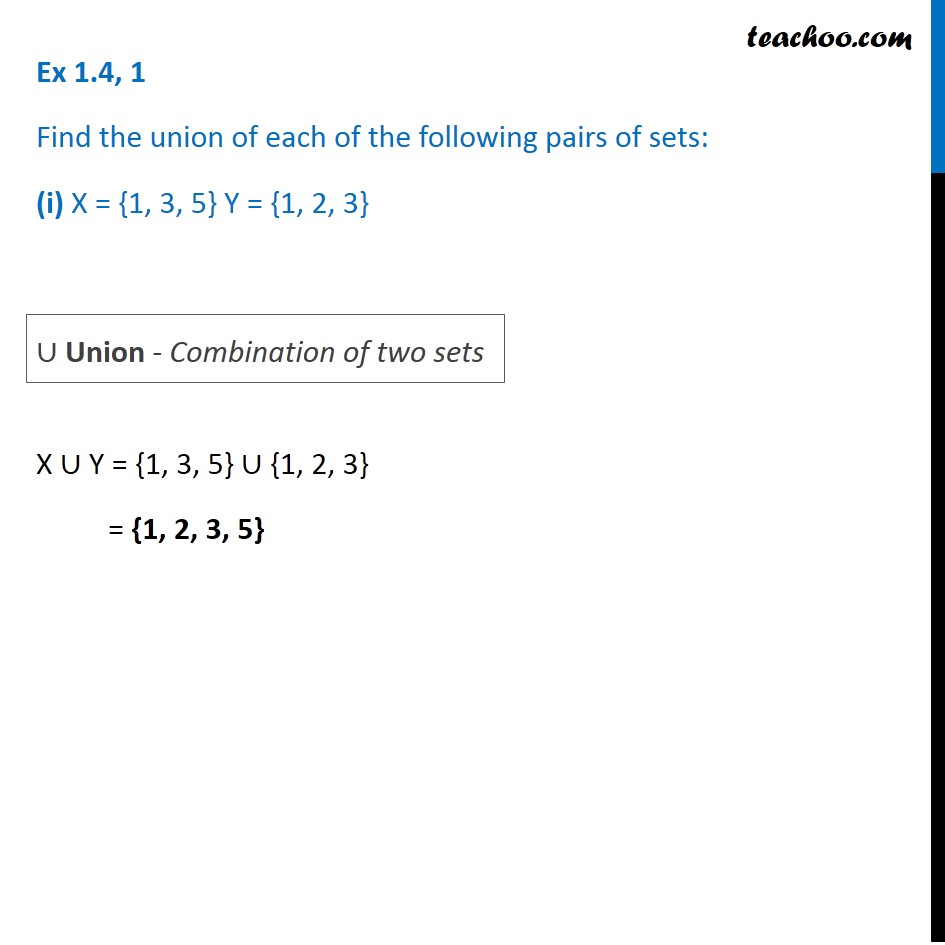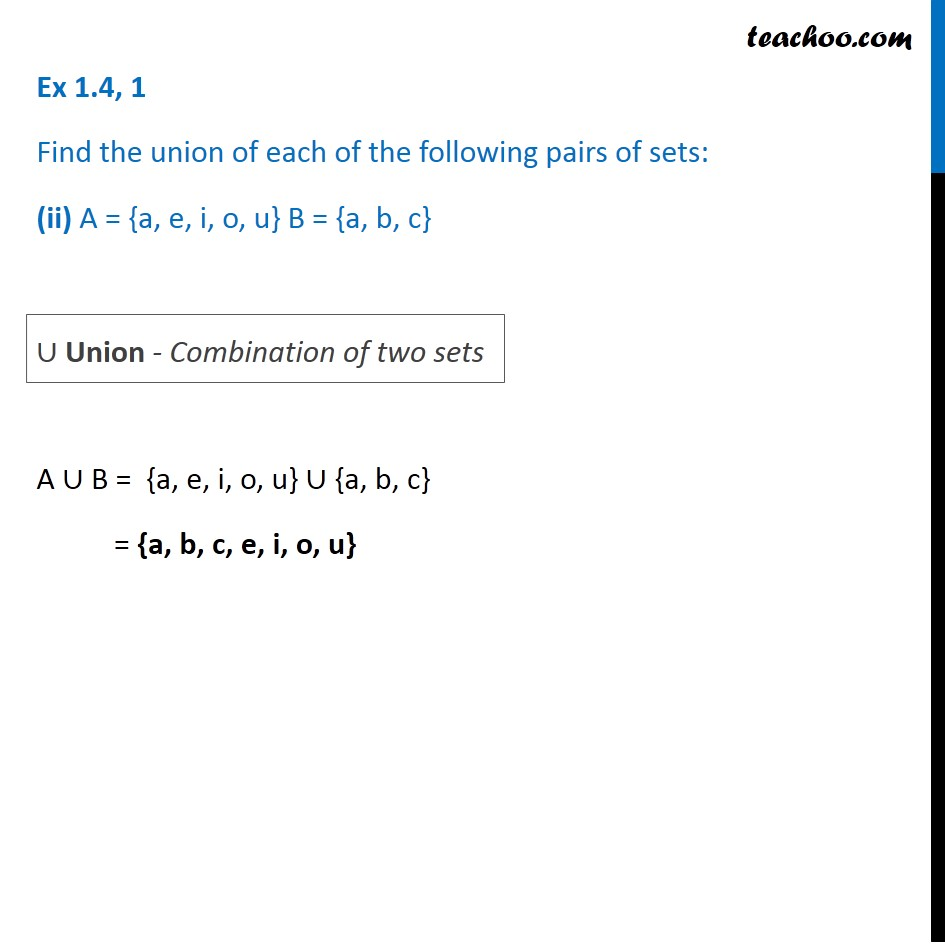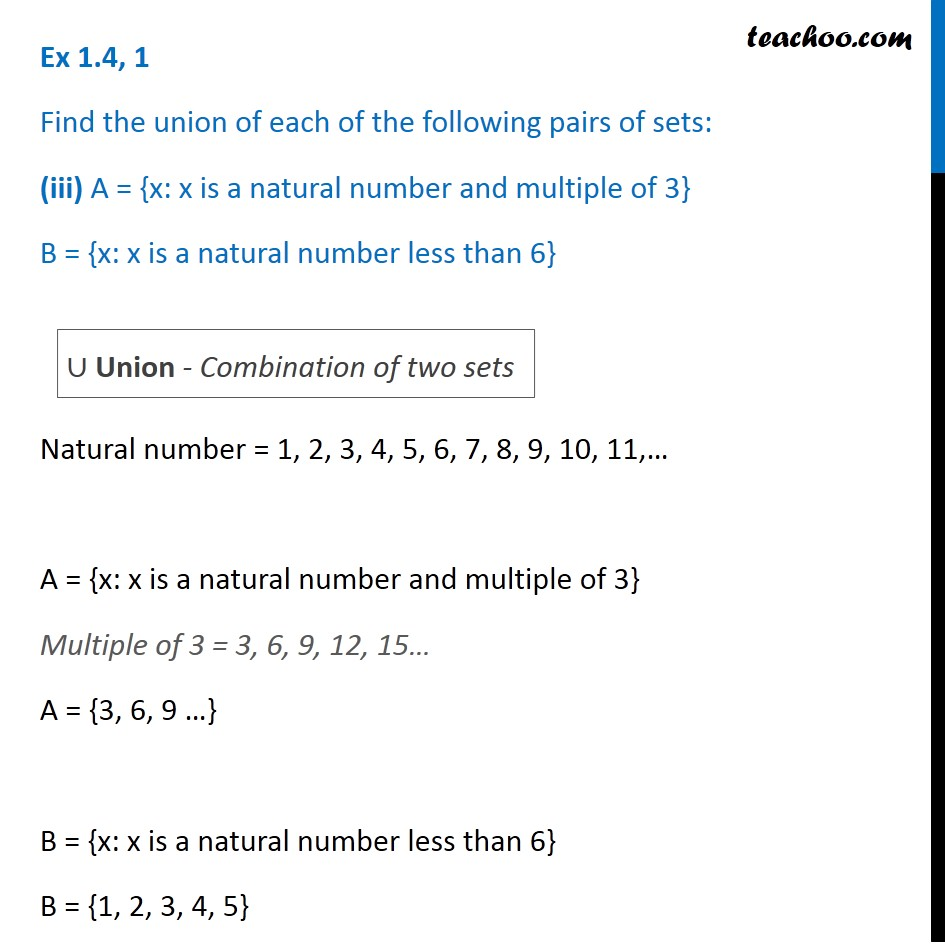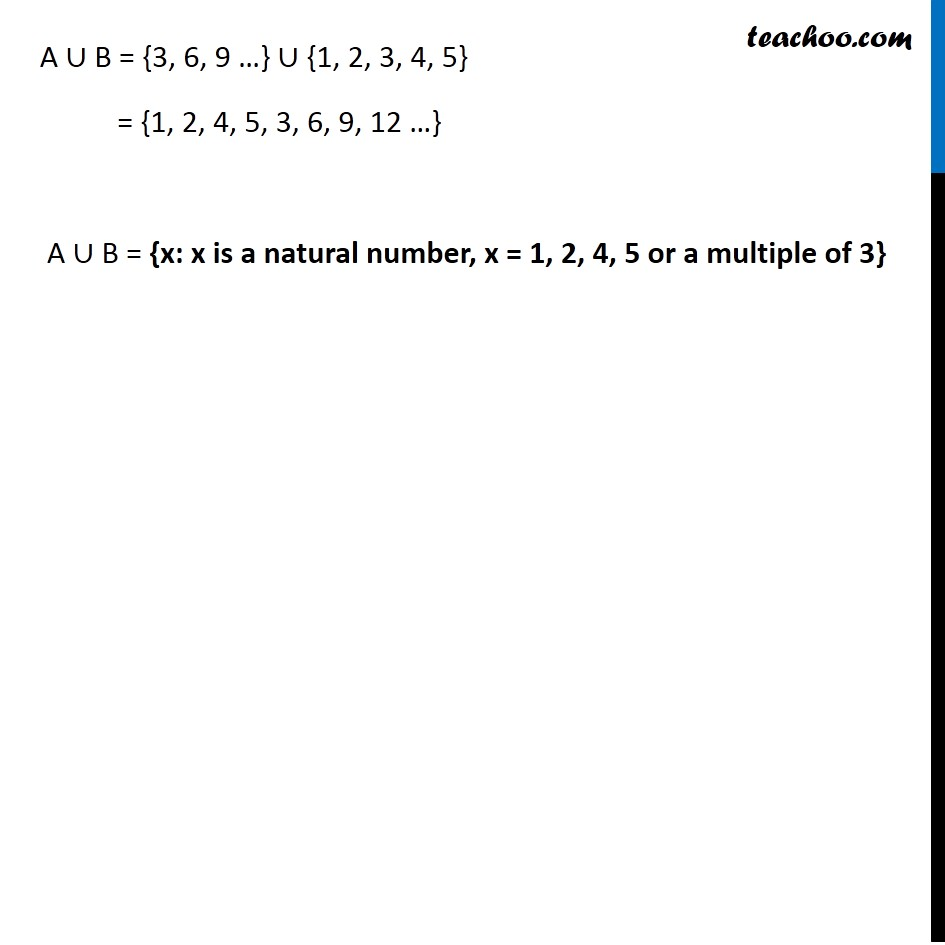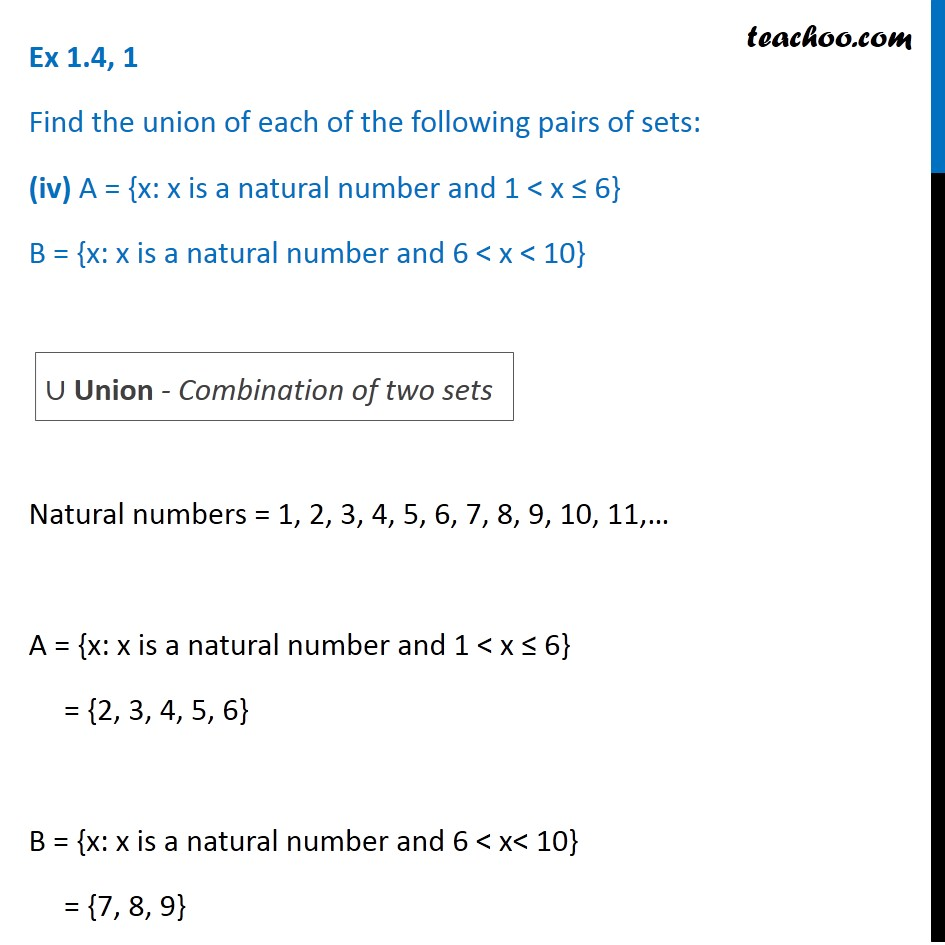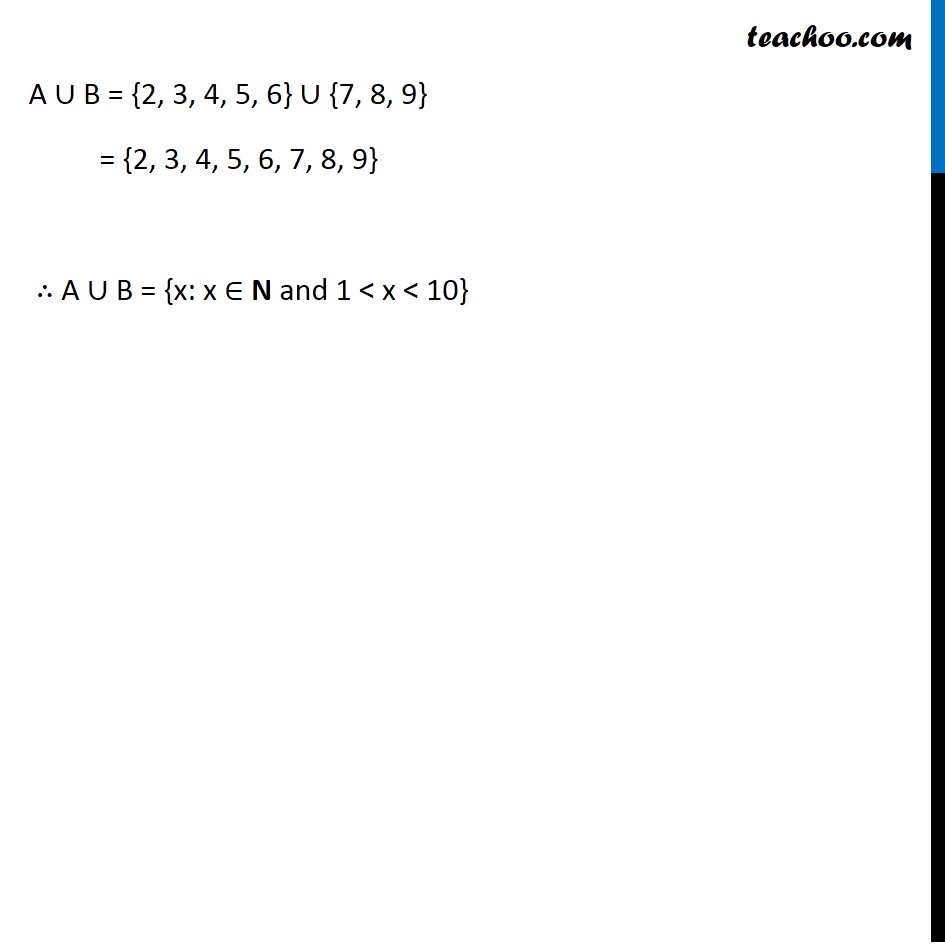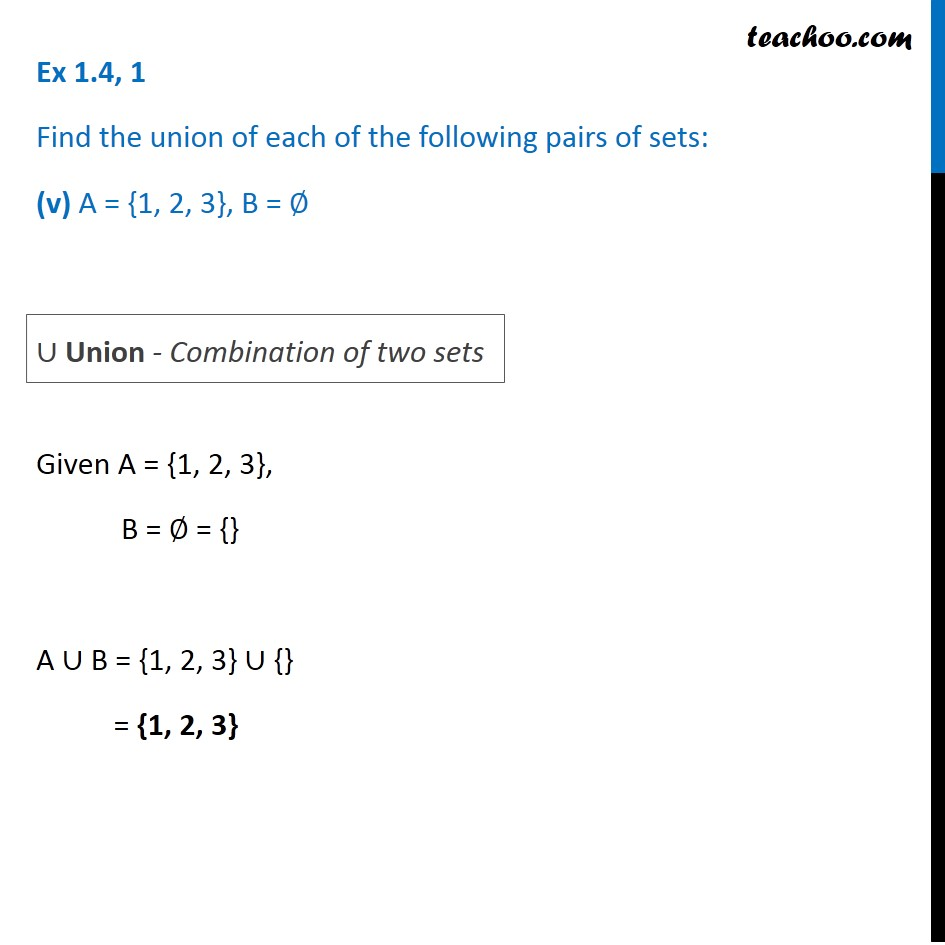1. Chapter 1 Class 11 Sets (Term 1)
2. Serial order wise
3. Ex 1.4

Transcript

Ex 1.4, 1 Find the union of each of the following pairs of sets: (i) X = {1, 3, 5} Y = {1, 2, 3} X ∪ Y = {1, 3, 5} ∪ {1, 2, 3} = {1, 2, 3, 5} ∪ Union - Combination of two sets Ex 1.4, 1 Find the union of each of the following pairs of sets: (ii) A = {a, e, i, o, u} B = {a, b, c} A ∪ B = {a, e, i, o, u} ∪ {a, b, c} = {a, b, c, e, i, o, u} ∪ Union - Combination of two sets Ex 1.4, 1 Find the union of each of the following pairs of sets: (iii) A = {x: x is a natural number and multiple of 3} B = {x: x is a natural number less than 6} Natural number = 1, 2, 3, 4, 5, 6, 7, 8, 9, 10, 11,… A = {x: x is a natural number and multiple of 3} Multiple of 3 = 3, 6, 9, 12, 15… A = {3, 6, 9 …} B = {x: x is a natural number less than 6} B = {1, 2, 3, 4, 5} ∪ Union - Combination of two sets A ∪ B = {3, 6, 9 …} ∪ {1, 2, 3, 4, 5} = {1, 2, 4, 5, 3, 6, 9, 12 …} A ∪ B = {x: x is a natural number, x = 1, 2, 4, 5 or a multiple of 3} Ex 1.4, 1 Find the union of each of the following pairs of sets: (iv) A = {x: x is a natural number and 1 < x ≤ 6} B = {x: x is a natural number and 6 < x < 10} Natural numbers = 1, 2, 3, 4, 5, 6, 7, 8, 9, 10, 11,… A = {x: x is a natural number and 1 < x ≤ 6} = {2, 3, 4, 5, 6} B = {x: x is a natural number and 6 < x< 10} = {7, 8, 9} ∪ Union - Combination of two sets A ∪ B = {2, 3, 4, 5, 6} ∪ {7, 8, 9} = {2, 3, 4, 5, 6, 7, 8, 9} ∴ A ∪ B = {x: x ∈ N and 1 < x < 10} Ex 1.4, 1 Find the union of each of the following pairs of sets: (v) A = {1, 2, 3}, B = ∅ Given A = {1, 2, 3}, B = ∅ = {} A ∪ B = {1, 2, 3} ∪ {} = {1, 2, 3} ∪ Union - Combination of two sets

Ex 1.4